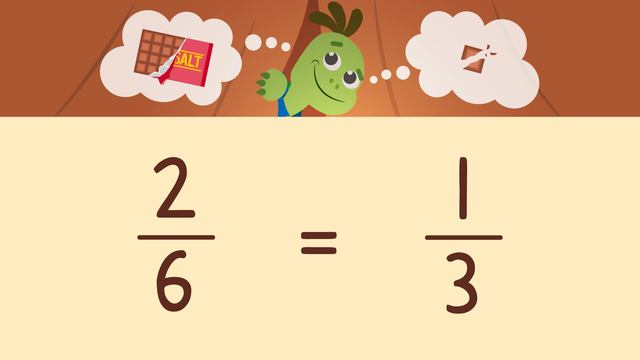# Simplifying FractionsRate this video

Ø 5.0 / 1 ratings

The authorTeam Digital
Simplifying Fractions
CCSS.MATH.CONTENT.4.NF.A.1

## Information about the videoSimplifying Fractions

### Contents

#### In this Video

In this simplifying fractions video, Tank wants a part of his favorite chocolate bar, Succulent Sea Salt. Axel offers to share if Tank can answer his riddle. In this simplifying fractions lesson, we will learn the simplifying fractions definition and the simplifying fractions steps to help Tank solve the riddle and share in a piece of his chocolate bar.

#### How to Simplify Fraction

How do you simplify fractions? We simplify a fraction by dividing the numerator and denominator by the same number. Why do we simplify fractions? We simplify fractions to create equivalent fractions with fewer parts to make them easier to understand.#### Simplifying Fractions Examples

How do you simplify fractions step by step? First, we want to think of a common factor that BOTH the numerator and denominator share. Next, we divide BOTH the numerator and denominator by that number.